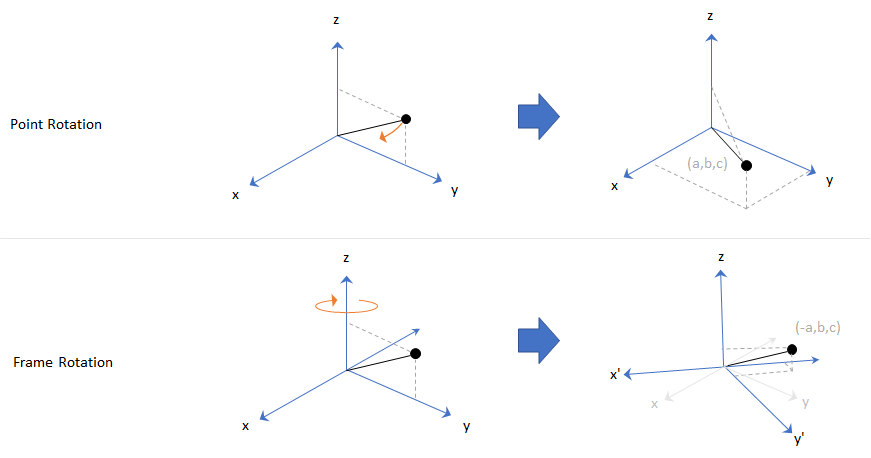# euler

Convert quaternion to Euler angles (radians)

Since R2021a

## Syntax

``eulerAngles = euler(quat,rotationSequence,rotationType)``

## Description

example

````eulerAngles = euler(quat,rotationSequence,rotationType)` converts the quaternion, `quat`, to an N-by-3 matrix of Euler angles.```

## Examples

collapse all

Convert a quaternion frame rotation to Euler angles in radians using the 'ZYX' rotation sequence.

```quat = quaternion([0.7071 0.7071 0 0]); eulerAnglesRandians = euler(quat,'ZYX','frame')```
```eulerAnglesRandians = 1×3 0 0 1.5708 ```

## Input Arguments

collapse all

Quaternion to convert to Euler angles, specified as a scalar, vector, matrix, or multidimensional array of quaternions.

Data Types: `quaternion`

Rotation sequence of Euler representation, specified as a character vector or string.

The rotation sequence defines the order of rotations about the axes. For example, if you specify a rotation sequence of `'YZX'`:

1. The first rotation is about the y-axis.

2. The second rotation is about the new z-axis.

3. The third rotation is about the new x-axis.

Data Types: `char` | `string`

Type of rotation, specified as `'point'` or `'frame'`.

In a point rotation, the frame is static and the point moves. In a frame rotation, the point is static and the frame moves. Point rotation and frame rotation define equivalent angular displacements but in opposite directions.Data Types: `char` | `string`

## Output Arguments

collapse all

Euler angle representation in radians, returned as a N-by-3 matrix. N is the number of quaternions in the `quat` argument.

For each row of `eulerAngles`, the first element corresponds to the first axis in the rotation sequence, the second element corresponds to the second axis in the rotation sequence, and the third element corresponds to the third axis in the rotation sequence.

The data type of the Euler angles representation is the same as the underlying data type of `quat`.

Data Types: `single` | `double`

## Version History

Introduced in R2021a